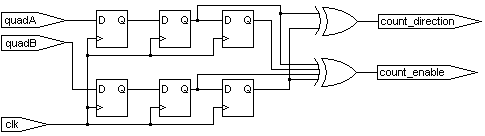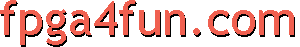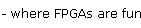FPGAs are suitable to create quadrature decoders.

Quadrature signals are two signals generated with a 90 degrees phase difference. They are used in mechanical systems to determine movement (or rotation) of an axis.

Here's one axis moving forward by a few steps.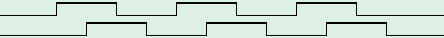If you count the pulses, you can say that the axis moved by 3 steps.
If you count the edges, you can say that the axis moved by 12 steps. That's what we do on this page.

Now the axis is moving backward by the same amount.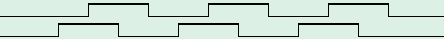So the idea is that by looking at the edges and levels, we can determine the direction and distance of movement.
Here's an example where an axis moves forward 10 steps, then backward 7 steps.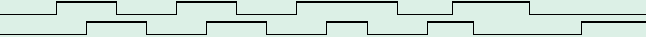#### Where are they used?

• In robotic axles, for feedback control.
• With knobs, to determine user input.
• In computer mice, to determine the direction of movement.

If you open a mechanical mouse, here's what you can see.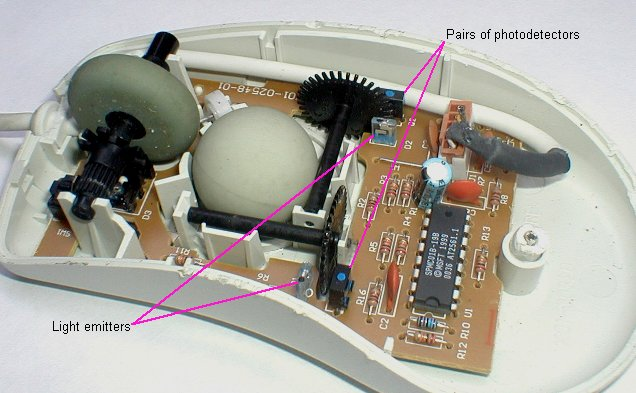There are two optical quadrature encoders, each made from a slotted wheel, a light emitter and a pair of photodetectors.

The mouse includes an IC responsible for the quadrature decoding and the serial/PS2 interface. Since it is easier to create a quadrature decoder (in an FPGA) than a serial or PS2 interface, we modified the mouse and replaced the original IC with a quad-buffers Schmitt trigger inputs IC.We used a CD4093 with the inputs of the each NAND gate tied together to form inverters.
Now the mouse outputs a quadrature encoded signal!

We want to implement a counter that increments or decrements according to the quadrature signals. We assume that we have available an "oversampling clock" (named "clk" in this page) that is faster than the quadrature signals.

The hardware circuit that controls the counter is surprisingly simple to do.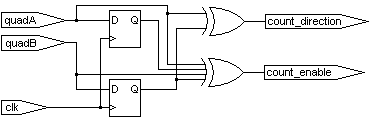Here's a waveform where an axis moves in forward direction, so that the counter increments.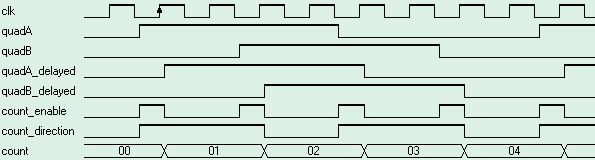This circuit is sometimes called a "4x decoder" because it counts all the transitions of the quadrature inputs.

In verilog HDL, that gives us:

#### Real life circuit

The previous circuit assumed that the "quadX" inputs were synchronous to the "clk" clock. In most cases, the "quadX" signals are not synchronous to the FPGA clock. The classical solution is to use 2 extra D flip-flops per input to avoid introducing metastability into the counter.